Share filterDeveloped by in Education category

4/5.0 Ratings

5,000 - 10,000 Installs

73 Reviews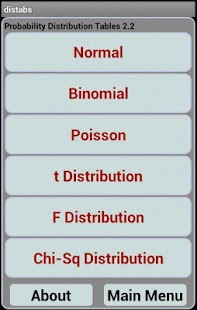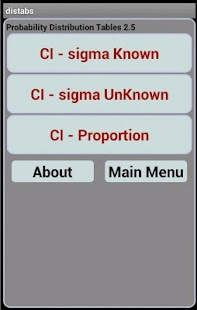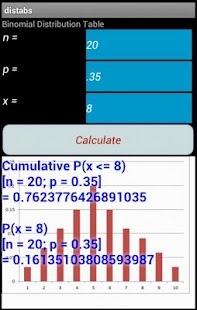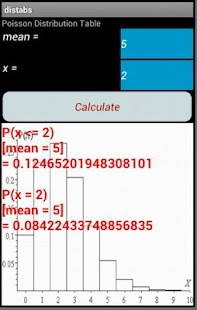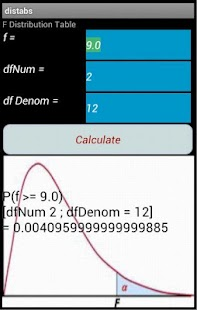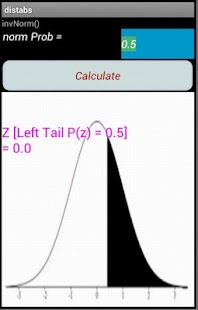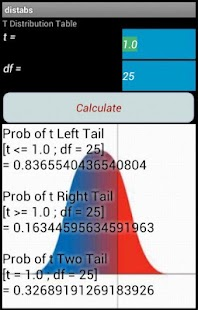Description

Version 2.6
Add Confidence Interval Calculators.
Improved display tablet landscape.
Chi Square Distribution Added.
Improved readability and formatting.
Improved menu system and labels.

Convenient, Accurate, Easy to Read
electronic Probability Distribution Tables.

Normal, Binomial, Poisson, T and F
Distributions. Also, invNorm and
invT calculations.

Now contains calculators for Confidence
Intervals (Sigma Known, Sigma Unknown,
Proportion).

Great for Intro Probability Statistics
courses, or if you just need these tables!

For both Phone and 10' tablets.

These tables give you the probabilities
associated with your test statistics and
parameters.

To use these tables, just select the
distribution you want, and put in the
test statistic and parameters, and the
APP tells you the related probability.

*************************************

1) For the Normal Distribution:

Enter your Z Score, and you get
the left, right tails and the range.

2) For the Binomial Distribution:

Enter n - number of trials,
p - probability of success,
x - number of successes

and you get the probability of
x, or the cumulative probability
for x.

3) For the Poisson Distribution:

Enter the mean, and number of
occurences, and you will get
the Probability of the number
of occurences, and the cumulative
probability.

4) For the t Distribution:

Enter t and the Degrees of Freedom
and you will get the probability
of the left and right tails, and
the two tailed probability.

5) For the f Distribution:

Enter the f statistic, and the
Degrees of Freedom for the numerator
and denominator. You will get the
probability for the right tail
(alpha for the ANOVA test).

6) For the Chi-Square Distribution:

Enter Chi-Square and the Degrees of Freedom
and you will get the probability
of the right tail.

7) Inverse Normal.

Enter the probability of the
Normal Distribution left tail,
and you get the Z statistic.

8) Inverse t

Enter the probability of the
t distribution left tail, along with
the degrees of freedom, and you will
get the t statistic.

Confidence Intervals:

Sigma Known. Uses the standard
CI formula (Using Z) to determine the range with
CI% chance of containing the true mean
of the population.

Sigma unKnown. Uses the standard
CI formula (Using t) to determine the range with
CI% chance of containing the true mean
of the population.

CI for a proportion. Uses the standard
CI formula (Using p,n,Z) to determine the range with
CI% chance of containing the true mean
of the population.

Free, but please visit this APP in
the Google Play store to Rate and
enter comments. This will help us
improve the APP over time.

Keywords: statistics statistical tables
probability distribution confidence interval

This content has been crawled through Play Store, we don't store any APK file.